Nuclear Decay and Conservation Laws

# Nuclear Decay and Conservation Laws - Notes | Study Modern Physics for IIT JAM - Physics

 1 Crore+ students have signed up on EduRev. Have you?

Nuclear decay has provided an amazing window into the realm of the very small. Nuclear decay gave the first indication of the connection between mass and energy, and it revealed the existence of two of the four basic forces in nature. In this section, we explore the major modes of nuclear decay; and, like those who first explored them, we will discover evidence of previously unknown particles and conservation laws.
Some nuclides are stable, apparently living forever. Unstable nuclides decay (that is, they are radioactive), eventually producing a stable nuclide after many decays. We call the original nuclide the parent and its decay products the daughters. Some radioactive nuclides decay in a single step to a stable nucleus. For example, 60Co is unstable and decays directly to 60Ni, which is stable. Others, such as 238U, decay to another unstable nuclide, resulting in a decay series in which each subsequent nuclide decays until a stable nuclide is finally produced. The decay series that starts from 238U is of particular interest, since it produces the radioactive isotopes 226Ra and 210Po, which the Curies first discovered. Radon gas is also produced (222Rn in the series), an increasingly recognized naturally occurring hazard. Since radon is a noble gas, it emanates from materials, such as soil, containing even trace amounts of 238U and can be inhaled. The decay of radon and its daughters produces internal damage. The 238U decay series ends with 206Pb, a stable isotope of lead.
The decay series produced by 238U, the most common uranium isotope. Nuclides are graphed in the same manner as in the chart of nuclides. The type of decay for each member of the series is shown, as well as the half-lives. Note that some nuclides decay by more than one mode. You can see why radium and polonium are found in uranium ore. A stable isotope of lead is the end product of the series.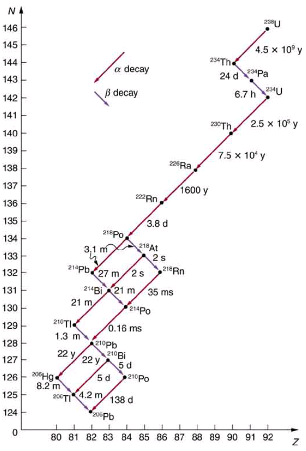Note that the daughters of α decay shown in always have two fewer protons and two fewer neutrons than the parent. This seems reasonable, since we know that α decay is the emission of a 4He nucleus, which has two protons and two neutrons. The daughters of β decay have one less neutron and one more proton than their parent. Beta decay is a little more subtle, as we shall see. No γ decays are shown in the figure, because they do not produce a daughter that differs from the parent.
Alpha Decay
In alpha decay, a 4He nucleus simply breaks away from the parent nucleus, leaving a daughter with two fewer protons and two fewer neutrons than the parent. One example of α decay is shown in for 238U. Another nuclide that undergoes α decay is 239Pu. The decay equations for these two nuclides are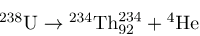and
239Pu → 235U + 4He.
Alpha decay is the separation of a 4He nucleus from the parent. The daughter nucleus has two fewer protons and two fewer neutrons than the parent. Alpha decay occurs spontaneously only if the daughter and  4He  nucleus have less total mass than the parent.If you examine the periodic table of the elements, you will find that Th has Z=90, two fewer than U, which has Z=92. Similarly, in the second decay equation, we see that U has two fewer protons than Pu, which has Z=94. The general rule for α decay is best written in the format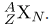If a certain nuclide is known to α decay (generally this information must be looked up in a table of isotopes, such as in Appendix B), its α decay equation is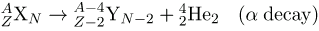where Y is the nuclide that has two fewer protons than X, such as Th having two fewer than U. So if you were told that 239Pu α decays and were asked to write the complete decay equation, you would first look up which element has two fewer protons (an atomic number two lower) and find that this is uranium. Then since four nucleons have broken away from the original 239, its atomic mass would be 235.
It is instructive to examine conservation laws related to α decay. You can see from the equation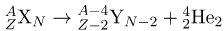that total charge is conserved. Linear and angular momentum are conserved, too. Although conserved angular momentum is not of great consequence in this type of decay, conservation of linear momentum has interesting consequences. If the nucleus is at rest when it decays, its momentum is zero. In that case, the fragments must fly in opposite directions with equal-magnitude momenta so that total momentum remains zero. This results in the α particle carrying away most of the energy, as a bullet from a heavy rifle carries away most of the energy of the powder burned to shoot it. Total mass–energy is also conserved: the energy produced in the decay comes from conversion of a fraction of the original mass. As discussed in Atomic Physics, the general relationship is
E = (Δm)c2.
Here, E is the nuclear reaction energy (the reaction can be nuclear decay or any other reaction), and Δm is the difference in mass between initial and final products. When the final products have less total mass, Δm is positive, and the reaction releases energy (is exothermic). When the products have greater total mass, the reaction is endothermic (Δm is negative) and must be induced with an energy input. For α decay to be spontaneous, the decay products must have smaller mass than the parent.
Alpha Decay Energy Found from Nuclear Masses
Find the energy emitted in the α decay of 239Pu.
Strategy
Nuclear reaction energy, such as released in α decay, can be found using the equation E=(Δm)c2. We must first find Δ m, the difference in mass between the parent nucleus and the products of the decay. This is easily done using masses given in Appendix A.
Solution
The decay equation was given earlier for 239Pu ; it is
239Pu → 235U + 4He.
Thus the pertinent masses are those of 239Pu, 235U, and the α particle or 4He, all of which are listed in Appendix A. The initial mass was m(239Pu) = 239.052157 u. The final mass is the sum
m(235U) + m(4He) = 239.043924 u + 4.002602 u = 239.046526 u. Thus,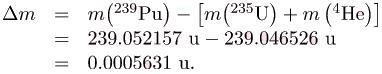Now we can find E by entering Δm into the equation:
E = (Δm)c= (0.005631 u)c2.
We know 1 u = 931.5 MeVc2, and so
E =  (0.005631) (931.5 MeV/c2)(c2)=5.25 MeV.
Discussion
The energy released in this α decay is in the MeV range, about 106 times as great as typical chemical reaction energies, consistent with many previous discussions. Most of this energy becomes kinetic energy of the α particle (or 4He nucleus), which moves away at high speed. The energy carried away by the recoil of the 235U nucleus is much smaller in order to conserve momentum. The 235U nucleus can be left in an excited state to later emit photons (Δ rays). This decay is spontaneous and releases energy, because the products have less mass than the parent nucleus. The question of why the products have less mass will be discussed in Binding Energy. Note that the masses given in Appendix A are atomic masses of neutral atoms, including their electrons. The mass of the electrons is the same before and after α decay, and so their masses subtract out when finding Δm. In this case, there are 94 electrons before and after the decay.
Beta Decay
There are actually three types of beta decay. The first discovered was “ordinary” beta decay and is called β- decay or electron emission. The symbol β- represents an electron emitted in nuclear beta decay. Cobalt-60 is a nuclide that β-  decays in the following manner:
60Co → 60Ni + β + neutrino.
The neutrino is a particle emitted in beta decay that was unanticipated and is of fundamental importance. The neutrino was not even proposed in theory until more than 20 years after beta decay was known to involve electron emissions. Neutrinos are so difficult to detect that the first direct evidence of them was not obtained until 1953. Neutrinos are nearly massless, have no charge, and do not interact with nucleons via the strong nuclear force. Traveling approximately at the speed of light, they have little time to affect any nucleus they encounter. This is, owing to the fact that they have no charge (and they are not EM waves), they do not interact through the EM force. They do interact via the relatively weak and very short range weak nuclear force. Consequently, neutrinos escape almost any detector and penetrate almost any shielding. However, neutrinos do carry energy, angular momentum (they are fermions with half-integral spin), and linear momentum away from a beta decay. When accurate measurements of beta decay were made, it became apparent that energy, angular momentum, and linear momentum were not accounted for by the daughter nucleus and electron alone. Either a previously unsuspected particle was carrying them away, or three conservation laws were being violated. Wolfgang Pauli made a formal proposal for the existence of neutrinos in 1930. The Italian-born American physicist Enrico Fermi (1901–1954) gave neutrinos their name, meaning little neutral ones, when he developed a sophisticated theory of beta decay (see . Part of Fermi’s theory was the identification of the weak nuclear force as being distinct from the strong nuclear force and in fact responsible for beta decay.
Enrico Fermi was nearly unique among 20th-century physicists—he made significant contributions both as an experimentalist and a theorist. His many contributions to theoretical physics included the identification of the weak nuclear force. The fermi (fm) is named after him, as are an entire class of subatomic particles (fermions), an element (Fermium), and a major research laboratory (Fermilab). His experimental work included studies of radioactivity, for which he won the 1938 Nobel Prize in physics, and creation of the first nuclear chain reaction. (credit: United States Department of Energy, Office of Public Affairs)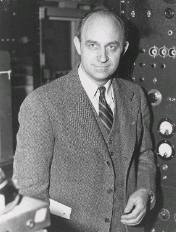The neutrino also reveals a new conservation law. There are various families of particles, one of which is the electron family. We propose that the number of members of the electron family is constant in any process or any closed system. In our example of beta decay, there are no members of the electron family present before the decay, but after, there is an electron and a neutrino. So electrons are given an electron family number of +1. The neutrino in β- decay is an electron’s antineutrino, given the symbol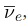where ν is the Greek letter nu, and the subscript e means this neutrino is related to the electron. The bar indicates this is a particle of antimatter. (All particles have antimatter counterparts that are nearly identical except that they have the opposite charge. Antimatter is almost entirely absent on Earth, but it is found in nuclear decay and other nuclear and particle reactions as well as in outer space.) The electron’s antineutrino, being antimatter, has an electron family number of -1. The total is zero, before and after the decay. The new conservation law, obeyed in all circumstances, states that the total electron family number is constant. An electron cannot be created without also creating an antimatter family member. This law is analogous to the conservation of charge in a situation where total charge is originally zero, and equal amounts of positive and negative charge must be created in a reaction to keep the total zero.
If a nuclide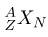is known to βdecay, then its β- decay equation is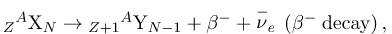where Y is the nuclide having one more proton than X. So if you know that a certain nuclide β- decays, you can find the daughter nucleus by first looking up
Z for the parent and then determining which element has atomic number
Z+1. In the example of the
β- decay of
60Co given earlier, we see that Z=27 for Co and
Z=28 is Ni. It is as if one of the neutrons in the parent nucleus decays into a proton, electron, and neutrino. In fact, neutrons outside of nuclei do just that—they live only an average of a few minutes and
β- decay in the following manner: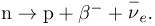In β- decay, the parent nucleus emits an electron and an antineutrino. The daughter nucleus has one more proton and one less neutron than its parent. Neutrinos interact so weakly that they are almost never directly observed, but they play a fundamental role in particle physics.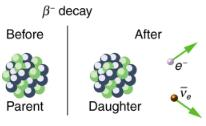We see that charge is conserved in β- decay, since the total charge is Z before and after the decay. For example, in 60Co decay, total charge is 27 before decay, since cobalt has
Z=27. After decay, the daughter nucleus is Ni, which has
Z=28, and there is an electron, so that the total charge is also 28 + (-1) or 27. Angular momentum is conserved, but not obviously (you have to examine the spins and angular momenta of the final products in detail to verify this). Linear momentum is also conserved, again imparting most of the decay energy to the electron and the antineutrino, since they are of low and zero mass, respectively. Another new conservation law is obeyed here and elsewhere in nature. The total number of nucleons A is conserved. In 60Co decay, for example, there are 60 nucleons before and after the decay. Note that total
A is also conserved in
α decay. Also note that the total number of protons changes, as does the total number of neutrons, so that total
Z and total N are not conserved in β- decay, as they are in α decay. Energy released in β- decay can be calculated given the masses of the parent and products.
β-Decay Energy from Masses
Find the energy emitted in the β- decay of 60Co.
Strategy and Concept
As in the preceding example, we must first find Δ m, the difference in mass between the parent nucleus and the products of the decay, using masses given in Appendix A. Then the emitted energy is calculated as before, using E=(Δm)c2. The initial mass is just that of the parent nucleus, and the final mass is that of the daughter nucleus and the electron created in the decay. The neutrino is massless, or nearly so. However, since the masses given in Appendix A are for neutral atoms, the daughter nucleus has one more electron than the parent, and so the extra electron mass that corresponds to the βis included in the atomic mass of Ni. Thus,
Δm = m(60Co) - m(60Ni).
Solution
The β- decay equation for 60Co is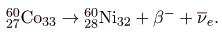As noticed,
Δm = m(60Co) - m(60Ni).
Entering the masses found in Appendix A gives
Δm = 59.933820 u - 59.930789 u = 0.003031 u.
Thus,
E = (Δm)c2 = (0.003031 u)c2.
Using 1 u=931.5 MeV/c2, we obtain
E = (0.003031) (931.5MeV/c2) (c2) = 2.82 MeV.
Discussion and Implications
Perhaps the most difficult thing about this example is convincing yourself that the β mass is included in the atomic mass of 60Ni. Beyond that are other implications. Again the decay energy is in the MeV range. This energy is shared by all of the products of the decay. In many 60Co decays, the daughter nucleus 60Ni is left in an excited state and emits photons (γ rays). Most of the remaining energy goes to the electron and neutrino, since the recoil kinetic energy of the daughter nucleus is small. One final note: the electron emitted in β- decay is created in the nucleus at the time of decay.
The second type of beta decay is less common than the first. It is β+decay. Certain nuclides decay by the emission of a positive electron. This is antielectron or positron decay.
βdecay is the emission of a positron that eventually finds an electron to annihilate, characteristically producing gammas in opposite directions.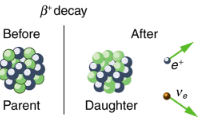The antielectron is often represented by the symbol e+, but in beta decay it is written as β+ to indicate the antielectron was emitted in a nuclear decay. Antielectrons are the antimatter counterpart to electrons, being nearly identical, having the same mass, spin, and so on, but having a positive charge and an electron family number of -1. When a positron encounters an electron, there is a mutual annihilation in which all the mass of the antielectron-electron pair is converted into pure photon energy. (The reaction, e++e-→ to γ + γ, conserves electron family number as well as all other conserved quantities.) If a nuclide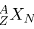is known to β+ decay, then its βdecay equation is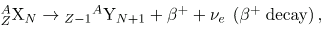where Y is the nuclide having one less proton than X (to conserve charge) and ve is the symbol for the electron’s neutrino, which has an electron family number of +1. Since an antimatter member of the electron family β+ is created in the decay, a matter member of the family (here the ve) must also be created. Given, for example, that
22Na
β+ decays, you can write its full decay equation by first finding that Z=11 for 22Na, so that the daughter nuclide will have
Z=10, the atomic number for neon. Thus the β+ decay equation for 22Na is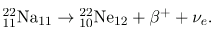In β+ decay, it is as if one of the protons in the parent nucleus decays into a neutron, a positron, and a neutrino. Protons do not do this outside of the nucleus, and so the decay is due to the complexities of the nuclear force. Note again that the total number of nucleons is constant in this and any other reaction. To find the energy emitted in βdecay, you must again count the number of electrons in the neutral atoms, since atomic masses are used. The daughter has one less electron than the parent, and one electron mass is created in the decay. Thus, in β+ decay,
Δm = m(parent) - [m(daugther) + 2me],
since we use the masses of neutral atoms.
Electron capture is the third type of beta decay. Here, a nucleus captures an inner-shell electron and undergoes a nuclear reaction that has the same effect as β+ decay. Electron capture is sometimes denoted by the letters EC. We know that electrons cannot reside in the nucleus, but this is a nuclear reaction that consumes the electron and occurs spontaneously only when the products have less mass than the parent plus the electron. If a nuclide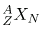is known to undergo electron capture, then its electron capture equation is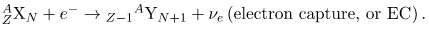Any nuclide that can β+ decay can also undergo electron capture (and often does both). The same conservation laws are obeyed for EC as for β+ decay. It is good practice to confirm these for yourself.
All forms of beta decay occur because the parent nuclide is unstable and lies outside the region of stability in the chart of nuclides. Those nuclides that have relatively more neutrons than those in the region of stability will βdecay to produce a daughter with fewer neutrons, producing a daughter nearer the region of stability. Similarly, those nuclides having relatively more protons than those in the region of stability will βdecay or undergo electron capture to produce a daughter with fewer protons, nearer the region of stability.
Gamma Decay
Gamma decay is the simplest form of nuclear decay—it is the emission of energetic photons by nuclei left in an excited state by some earlier process. Protons and neutrons in an excited nucleus are in higher orbitals, and they fall to lower levels by photon emission (analogous to electrons in excited atoms). Nuclear excited states have lifetimes typically of only about 10-14 s, an indication of the great strength of the forces pulling the nucleons to lower states. The γ decay equation is simply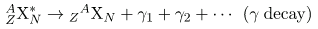where the asterisk indicates the nucleus is in an excited state. There may be one or more γ s emitted, depending on how the nuclide de-excites. In radioactive decay, γ emission is common and is preceded by γ or β decay. For example, when 60Co β- decays, it most often leaves the daughter nucleus in an excited state, written 60Ni*. Then the nickel nucleus quickly γ decays by the emission of two penetrating γ s: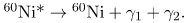These are called cobalt γ rays, although they come from nickel—they are used for cancer therapy, for example. It is again constructive to verify the conservation laws for gamma decay. Finally, since γ decay does not change the nuclide to another species, it is not prominently featured in charts of decay series, such as that in.
There are other types of nuclear decay, but they occur less commonly than α,
β, and γ decay. Spontaneous fission is the most important of the other forms of nuclear decay because of its applications in nuclear power and weapons. It is covered in the next chapter.
Section Summary

• When a parent nucleus decays, it produces a daughter nucleus following rules and conservation laws. There are three major types of nuclear decay, called alpha (α), beta β (β), and gamma (γ). The α decay equation is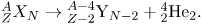• Nuclear decay releases an amount of energy E related to the mass destroyed Δm by
E = (Δm)c2.
• There are three forms of beta decay. The βdecay equation is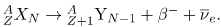• The β+ decay equation is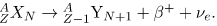• The electron capture equation is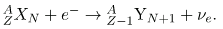• β is an electron, β+ is an antielectron or positron, ve represents an electron’s neutrino, and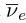is an electron’s antineutrino. In addition to all previously known conservation laws, two new ones arise— conservation of electron family number and conservation of the total number of nucleons. The γ decay equation is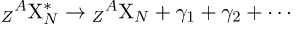γ is a high-energy photon originating in a nucleus.

Conceptual Questions

1. Star Trek fans have often heard the term “antimatter drive.” Describe how you could use a magnetic field to trap antimatter, such as produced by nuclear decay, and later combine it with matter to produce energy. Be specific about the type of antimatter, the need for vacuum storage, and the fraction of matter converted into energy.
2. What conservation law requires an electron’s neutrino to be produced in electron capture? Note that the electron no longer exists after it is captured by the nucleus.
3. Neutrinos are experimentally determined to have an extremely small mass. Huge numbers of neutrinos are created in a supernova at the same time as massive amounts of light are first produced. When the 1987A supernova occurred in the Large Magellanic Cloud, visible primarily in the Southern Hemisphere and some 100,000 light-years away from Earth, neutrinos from the explosion were observed at about the same time as the light from the blast. How could the relative arrival times of neutrinos and light be used to place limits on the mass of neutrinos?
4. What do the three types of beta decay have in common that is distinctly different from alpha decay?

Problems & Exercises
In the following eight problems, write the complete decay equation for the given nuclide in the complete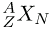notation. Refer to the periodic table for values of Z.
β- decay of 3H (tritium), a manufactured isotope of hydrogen used in some digital watch displays, and manufactured primarily for use in hydrogen bombs.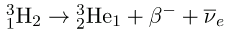βdecay of 40K, a naturally occurring rare isotope of potassium responsible for some of our
β+ decay of  50Mn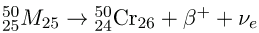β+ decay of  52Ee.
Electron capture by 7Be.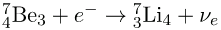Electron capture by 106In.
α decay of 210Po, the isotope of polonium in the decay series of 238U that was discovered by the Curies. A favorite isotope in physics labs, since it has a short half-life and decays to a stable nuclide.
In the following four problems, identify the parent nuclide and write the complete decay equation in the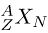notation. Refer to the periodic table for values of Z.
β-  decay producing 137Ba. The parent nuclide is a major waste product of reactors and has chemistry similar to potassium and sodium, resulting in its concentration in your cells if ingested.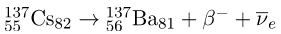βdecay producing 90Y. The parent nuclide is a major waste product of reactors and has chemistry similar to calcium, so that it is concentrated in bones if ingested (90Y is also radioactive.)
α decay producing 228Ra. The parent nuclide is nearly 100% of the natural element and is found in gas lantern mantles and in metal alloys used in jets (228Ra is also radioactive).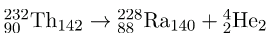α decay producing 208Pb. The parent nuclide is in the decay series produced by 232Th, the only naturally occurring isotope of thorium.
When an electron and positron annihilate, both their masses are destroyed, creating two equal energy photons to preserve momentum.
(a) Confirm that the annihilation equation e++ e→ γ + γ conserves charge, electron family number, and total number of nucleons. To do this, identify the values of each before and after the annihilation.
(b) Find the energy of each γ ray, assuming the electron and positron are initially nearly at rest.
(c) Explain why the two γ rays travel in exactly opposite directions if the center of mass of the electron-positron system is initially at rest.
charge: (+1) + (-1) = 0; electron family number: (+1) + (-1) = 0; A : (a)
(a) 0 + 0 = 0
(b) 0.511 MeV
(c) The two γ rays must travel in exactly opposite directions in order to conserve momentum, since initially there is zero momentum if the center of mass is initially at rest.
Confirm that charge, electron family number, and the total number of nucleons are all conserved by the rule for α decay given in the equation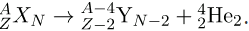To do this, identify the values of each before and after the decay.
Confirm that charge, electron family number, and the total number of nucleons are all conserved by the rule for
βdecay given in the equation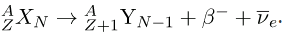To do this, identify the values of each before and after the decay.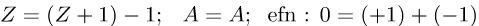Confirm that charge, electron family number, and the total number of nucleons are all conserved by the rule for β- decay given in the equation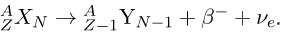To do this, identify the values of each before and after the decay.
Confirm that charge, electron family number, and the total number of nucleons are all conserved by the rule for electron capture given in the equation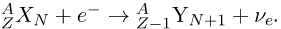To do this, identify the values of each before and after the capture.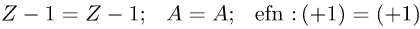A rare decay mode has been observed in which 222Ra emits a
14C nucleus.
(a) The decay equation is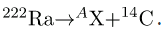Identify the nuclide Ax.
(b)  Find the energy emitted in the decay. The mass of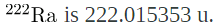(a) Write the complete α decay equation for 222Ra.
(b) Find the energy released in the decay.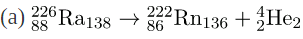(b) 4.87 MeV
(a) Write the complete α decay equation for 249Cf.
(b) Find the energy released in the decay.
(a) Write the complete βdecay equation for the neutron.
(b) Find the energy released in the decay.
(a) n → p + β- +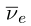(b) ) 0.783 MeV
(a) Write the complete β-  decay equation for 90Sr, a major waste product of nuclear reactors.
(b) Find the energy released in the decay.
Calculate the energy released in the β+ decay of 22Na, the equation for which is given in the text. The masses of
22Na and
22Ne are 21.994434 and 21.991383 u, respectively.
1.82 MeV
(a) Write the complete β+ decay equation for 11C.
(b) Calculate the energy released in the decay. The masses of 11C and 11B are 11.011433 and 11.009305 u, respectively.
(a) Calculate the energy released in the α decay of 238U.
(b) What fraction of the mass of a single 238U is destroyed in the decay? The mass of 234Th is 234.043593 u.
(c) Although the fractional mass loss is large for a single nucleus, it is difficult to observe for an entire macroscopic sample of uranium. Why is this?
(a) 4.274 MeV
1.92710-5
(c) Since U-238 is a slowly decaying substance, only a very small number of nuclei decay on human timescales; therefore, although those nuclei that decay lose a noticeable fraction of their mass, the change in the total mass of the sample is not detectable for a macroscopic sample.
(a) Write the complete reaction equation for electron capture by 7Be.
(b) Calculate the energy released.
(a) Write the complete reaction equation for electron capture by 15O.
(b) Calculate the energy released.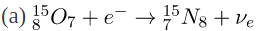(b) 2.754 MeV

The document Nuclear Decay and Conservation Laws - Notes | Study Modern Physics for IIT JAM - Physics is a part of the Physics Course Modern Physics for IIT JAM.
All you need of Physics at this link: Physics

## Modern Physics for IIT JAM

54 videos|44 docs|15 tests
 Use Code STAYHOME200 and get INR 200 additional OFF

## Modern Physics for IIT JAM

54 videos|44 docs|15 tests

Track your progress, build streaks, highlight & save important lessons and more!

,

,

,

,

,

,

,

,

,

,

,

,

,

,

,

,

,

,

,

,

,

;